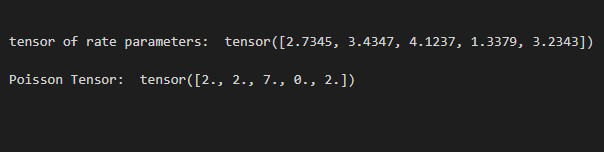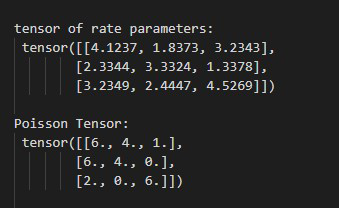# How to Create a Tensor Whose Elements are Sampled from a Poisson Distribution in PyTorch

• Last Updated : 02 Jun, 2022

In this article, we will discuss how to create a Tensor whose elements are Sampled from a Poisson Distribution in PyTorch in Python.

## torch.poisson() method

The torch.poisson() method helps us to create a tensor whose elements are sampled from a Poisson distribution. This method accepts a tensor as input and this input tensor contains the rates of the Poisson distribution. This method returns a tensor of the size same as the input tensor and the elements of this tensor are sampled from a Poisson distribution with the rate parameter. before moving further let’s see the syntax of the given method.

Syntax: torch.poisson(rates)

Parameters:

• rates (Tensor): This parameter is used to sample elements from a Poisson distribution.

Returns:This method returns a tensor and the elements of this tensor are sampled from a Poisson distribution with the rate parameter.

Example 1:

In this example, we will discuss how to create a 1D Tensor whose elements are Sampled from a Poisson Distribution.

## Python

 `# import required libraries``import` `torch`` ` `# create torch tensor of rate parameters``rates_tens ``=` `torch.tensor([``2.7345``, ``3.4347``,``                           ``4.1237``, ``1.3379``, ``3.2343``])``print``(``"tensor of rate parameters: "``, rates_tens)`` ` `# apply possion() method``pois_tens ``=` `torch.poisson(rates_tens)`` ` `# display result``print``(``"Poisson Tensor: "``, pois_tens)`

Output:Example 2: In this example, we will discuss how to create a 2D Tensor whose elements are Sampled from a Poisson Distribution.

## Python

 `# import required libraries``import` `torch`` ` `# create torch tensor of rate parameters``rates_tens ``=` `torch.tensor([[``4.1237``, ``1.8373``, ``3.2343``],``                           ``[``2.3344``, ``3.3324``, ``1.3378``],``                           ``[``3.2349``, ``2.4447``, ``4.5269``]])`` ` `print``(``"tensor of rate parameters: \n"``, rates_tens)`` ` `# apply possion() method``pois_tens ``=` `torch.poisson(rates_tens)`` ` `# display result``print``(``"Poisson Tensor: \n"``, pois_tens)`

Output:My Personal Notes arrow_drop_up